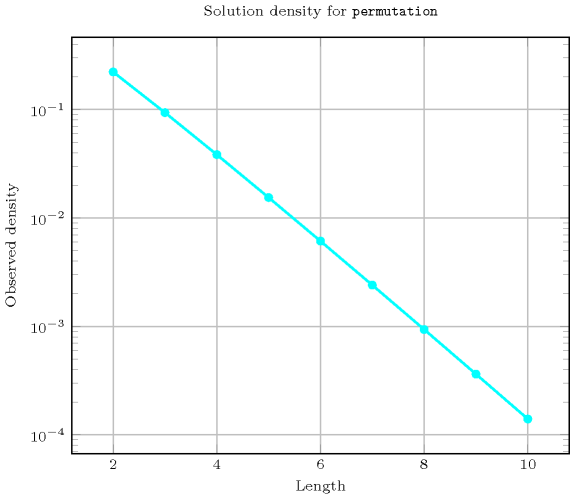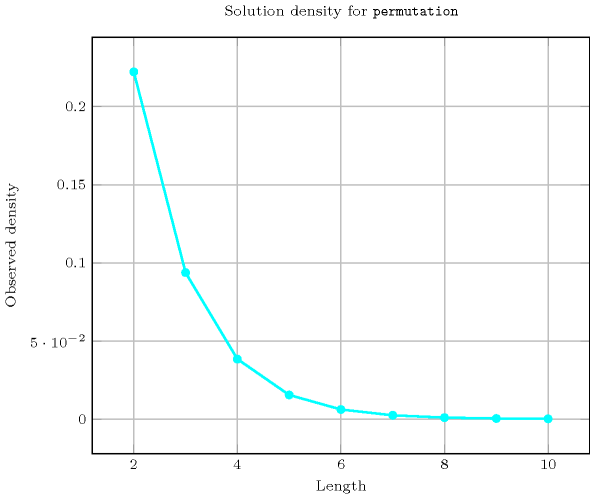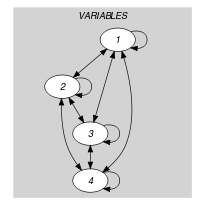## 5.322. permutation

Origin
Constraint

$\mathrm{𝚙𝚎𝚛𝚖𝚞𝚝𝚊𝚝𝚒𝚘𝚗}\left(\mathrm{𝚅𝙰𝚁𝙸𝙰𝙱𝙻𝙴𝚂}\right)$

Argument
 $\mathrm{𝚅𝙰𝚁𝙸𝙰𝙱𝙻𝙴𝚂}$ $\mathrm{𝚌𝚘𝚕𝚕𝚎𝚌𝚝𝚒𝚘𝚗}\left(\mathrm{𝚟𝚊𝚛}-\mathrm{𝚍𝚟𝚊𝚛}\right)$
Restrictions
 $\mathrm{𝚛𝚎𝚚𝚞𝚒𝚛𝚎𝚍}$$\left(\mathrm{𝚅𝙰𝚁𝙸𝙰𝙱𝙻𝙴𝚂},\mathrm{𝚟𝚊𝚛}\right)$ $\mathrm{𝚖𝚒𝚗𝚟𝚊𝚕}$$\left(\mathrm{𝚅𝙰𝚁𝙸𝙰𝙱𝙻𝙴𝚂}.\mathrm{𝚟𝚊𝚛}\right)=1$ $\mathrm{𝚖𝚊𝚡𝚟𝚊𝚕}$$\left(\mathrm{𝚅𝙰𝚁𝙸𝙰𝙱𝙻𝙴𝚂}.\mathrm{𝚟𝚊𝚛}\right)=|\mathrm{𝚅𝙰𝚁𝙸𝙰𝙱𝙻𝙴𝚂}|$
Purpose

Enforce all variables of the collection $\mathrm{𝚅𝙰𝚁𝙸𝙰𝙱𝙻𝙴𝚂}$ to take distinct values between 1 and the total number of variables.

Example
$\left(〈3,2,1,4〉\right)$

The $\mathrm{𝚙𝚎𝚛𝚖𝚞𝚝𝚊𝚝𝚒𝚘𝚗}$ constraint holds since all the values 3, 2, 1 and 4 are distinct, and since they all belong to interval $\left[1,4\right]$ where 4 is the total number of variables.

Typical
$|\mathrm{𝚅𝙰𝚁𝙸𝙰𝙱𝙻𝙴𝚂}|>2$
Symmetries
• Items of $\mathrm{𝚅𝙰𝚁𝙸𝙰𝙱𝙻𝙴𝚂}$ are permutable.

• Two distinct values of $\mathrm{𝚅𝙰𝚁𝙸𝙰𝙱𝙻𝙴𝚂}.\mathrm{𝚟𝚊𝚛}$ can be swapped.

Usage

See Usage slot of $\mathrm{𝚊𝚕𝚕𝚍𝚒𝚏𝚏𝚎𝚛𝚎𝚗𝚝}$.

Algorithm

See Algorithm slot of $\mathrm{𝚊𝚕𝚕𝚍𝚒𝚏𝚏𝚎𝚛𝚎𝚗𝚝}$.

Counting
 Length ($n$) 2 3 4 5 6 7 8 9 10 Solutions 2 6 24 120 720 5040 40320 362880 3628800

Number of solutions for $\mathrm{𝚙𝚎𝚛𝚖𝚞𝚝𝚊𝚝𝚒𝚘𝚗}$: domains $0..n$Keywords
Cond. implications

$•$ $\mathrm{𝚙𝚎𝚛𝚖𝚞𝚝𝚊𝚝𝚒𝚘𝚗}\left(\mathrm{𝚅𝙰𝚁𝙸𝙰𝙱𝙻𝙴𝚂}\right)$

implies $\mathrm{𝚋𝚊𝚕𝚊𝚗𝚌𝚎}$$\left(\mathrm{𝙱𝙰𝙻𝙰𝙽𝙲𝙴},\mathrm{𝚅𝙰𝚁𝙸𝙰𝙱𝙻𝙴𝚂}\right)$

when  $\mathrm{𝙱𝙰𝙻𝙰𝙽𝙲𝙴}=0$.

$•$ $\mathrm{𝚙𝚎𝚛𝚖𝚞𝚝𝚊𝚝𝚒𝚘𝚗}\left(\mathrm{𝚅𝙰𝚁𝙸𝙰𝙱𝙻𝙴𝚂}\right)$

implies $\mathrm{𝚌𝚑𝚊𝚗𝚐𝚎}$$\left(\mathrm{𝙽𝙲𝙷𝙰𝙽𝙶𝙴},\mathrm{𝚅𝙰𝚁𝙸𝙰𝙱𝙻𝙴𝚂},\mathrm{𝙲𝚃𝚁}\right)$

when  $\mathrm{𝙽𝙲𝙷𝙰𝙽𝙶𝙴}=|\mathrm{𝚅𝙰𝚁𝙸𝙰𝙱𝙻𝙴𝚂}|-1$

and   $\mathrm{𝙲𝚃𝚁}\in \left[\ne \right]$.

$•$ $\mathrm{𝚙𝚎𝚛𝚖𝚞𝚝𝚊𝚝𝚒𝚘𝚗}\left(\mathrm{𝚅𝙰𝚁𝙸𝙰𝙱𝙻𝙴𝚂}\right)$

implies $\mathrm{𝚌𝚒𝚛𝚌𝚞𝚕𝚊𝚛}_\mathrm{𝚌𝚑𝚊𝚗𝚐𝚎}$$\left(\mathrm{𝙽𝙲𝙷𝙰𝙽𝙶𝙴},\mathrm{𝚅𝙰𝚁𝙸𝙰𝙱𝙻𝙴𝚂},\mathrm{𝙲𝚃𝚁}\right)$

when  $\mathrm{𝙽𝙲𝙷𝙰𝙽𝙶𝙴}=|\mathrm{𝚅𝙰𝚁𝙸𝙰𝙱𝙻𝙴𝚂}|$

and   $\mathrm{𝙲𝚃𝚁}\in \left[\ne \right]$.

$•$ $\mathrm{𝚙𝚎𝚛𝚖𝚞𝚝𝚊𝚝𝚒𝚘𝚗}\left(\mathrm{𝚅𝙰𝚁𝙸𝙰𝙱𝙻𝙴𝚂}\right)$

implies $\mathrm{𝚕𝚎𝚗𝚐𝚝𝚑}_\mathrm{𝚕𝚊𝚜𝚝}_\mathrm{𝚜𝚎𝚚𝚞𝚎𝚗𝚌𝚎}$$\left(\mathrm{𝙻𝙴𝙽},\mathrm{𝚅𝙰𝚁𝙸𝙰𝙱𝙻𝙴𝚂}\right)$

when  $\mathrm{𝙻𝙴𝙽}=1$.

$•$ $\mathrm{𝚙𝚎𝚛𝚖𝚞𝚝𝚊𝚝𝚒𝚘𝚗}\left(\mathrm{𝚅𝙰𝚁𝙸𝙰𝙱𝙻𝙴𝚂}\right)$

implies $\mathrm{𝚕𝚎𝚗𝚐𝚝𝚑}_\mathrm{𝚏𝚒𝚛𝚜𝚝}_\mathrm{𝚜𝚎𝚚𝚞𝚎𝚗𝚌𝚎}$$\left(\mathrm{𝙻𝙴𝙽},\mathrm{𝚅𝙰𝚁𝙸𝙰𝙱𝙻𝙴𝚂}\right)$

when  $\mathrm{𝙻𝙴𝙽}=1$.

$•$ $\mathrm{𝚙𝚎𝚛𝚖𝚞𝚝𝚊𝚝𝚒𝚘𝚗}\left(\mathrm{𝚅𝙰𝚁𝙸𝙰𝙱𝙻𝙴𝚂}\right)$

implies $\mathrm{𝚕𝚘𝚗𝚐𝚎𝚜𝚝}_\mathrm{𝚌𝚑𝚊𝚗𝚐𝚎}$$\left(\mathrm{𝚂𝙸𝚉𝙴},\mathrm{𝚅𝙰𝚁𝙸𝙰𝙱𝙻𝙴𝚂},\mathrm{𝙲𝚃𝚁}\right)$

when  $\mathrm{𝚂𝙸𝚉𝙴}=|\mathrm{𝚅𝙰𝚁𝙸𝙰𝙱𝙻𝙴𝚂}|$

and   $\mathrm{𝙲𝚃𝚁}\in \left[\ne \right]$.

$•$ $\mathrm{𝚙𝚎𝚛𝚖𝚞𝚝𝚊𝚝𝚒𝚘𝚗}\left(\mathrm{𝚅𝙰𝚁𝙸𝙰𝙱𝙻𝙴𝚂}\right)$

implies $\mathrm{𝚖𝚊𝚡}_𝚗$$\left(\mathrm{𝙼𝙰𝚇},\mathrm{𝚁𝙰𝙽𝙺},\mathrm{𝚅𝙰𝚁𝙸𝙰𝙱𝙻𝙴𝚂}\right)$

when  $\mathrm{𝙼𝙰𝚇}=|\mathrm{𝚅𝙰𝚁𝙸𝙰𝙱𝙻𝙴𝚂}|-\mathrm{𝚁𝙰𝙽𝙺}$.

$•$ $\mathrm{𝚙𝚎𝚛𝚖𝚞𝚝𝚊𝚝𝚒𝚘𝚗}\left(\mathrm{𝚅𝙰𝚁𝙸𝙰𝙱𝙻𝙴𝚂}\right)$

implies $\mathrm{𝚖𝚒𝚗}_𝚗$$\left(\mathrm{𝙼𝙸𝙽},\mathrm{𝚁𝙰𝙽𝙺},\mathrm{𝚅𝙰𝚁𝙸𝙰𝙱𝙻𝙴𝚂}\right)$

when  $\mathrm{𝙼𝙸𝙽}=\mathrm{𝚁𝙰𝙽𝙺}+1$.

$•$ $\mathrm{𝚙𝚎𝚛𝚖𝚞𝚝𝚊𝚝𝚒𝚘𝚗}\left(\mathrm{𝚅𝙰𝚁𝙸𝙰𝙱𝙻𝙴𝚂}\right)$

implies $\mathrm{𝚖𝚒𝚗}_\mathrm{𝚗𝚟𝚊𝚕𝚞𝚎}$$\left(\mathrm{𝙼𝙸𝙽},\mathrm{𝚅𝙰𝚁𝙸𝙰𝙱𝙻𝙴𝚂}\right)$

when  $\mathrm{𝙼𝙸𝙽}=1$.

$•$ $\mathrm{𝚙𝚎𝚛𝚖𝚞𝚝𝚊𝚝𝚒𝚘𝚗}\left(\mathrm{𝚅𝙰𝚁𝙸𝙰𝙱𝙻𝙴𝚂}\right)$

implies $\mathrm{𝚖𝚒𝚗}_\mathrm{𝚜𝚒𝚣𝚎}_\mathrm{𝚏𝚞𝚕𝚕}_\mathrm{𝚣𝚎𝚛𝚘}_\mathrm{𝚜𝚝𝚛𝚎𝚝𝚌𝚑}$$\left(\mathrm{𝙼𝙸𝙽𝚂𝙸𝚉𝙴},\mathrm{𝚅𝙰𝚁𝙸𝙰𝙱𝙻𝙴𝚂}\right)$

when  $\mathrm{𝙼𝙸𝙽𝚂𝙸𝚉𝙴}=|\mathrm{𝚅𝙰𝚁𝙸𝙰𝙱𝙻𝙴𝚂}|$.

$•$ $\mathrm{𝚙𝚎𝚛𝚖𝚞𝚝𝚊𝚝𝚒𝚘𝚗}\left(\mathrm{𝚅𝙰𝚁𝙸𝙰𝙱𝙻𝙴𝚂}\right)$

implies $\mathrm{𝚗𝚒𝚗𝚝𝚎𝚛𝚟𝚊𝚕}$$\left(\mathrm{𝙽𝚅𝙰𝙻},\mathrm{𝚅𝙰𝚁𝙸𝙰𝙱𝙻𝙴𝚂},\mathrm{𝚂𝙸𝚉𝙴}_\mathrm{𝙸𝙽𝚃𝙴𝚁𝚅𝙰𝙻}\right)$

when  $\mathrm{𝙽𝚅𝙰𝙻}=\left(|\mathrm{𝚅𝙰𝚁𝙸𝙰𝙱𝙻𝙴𝚂}|+\mathrm{𝚂𝙸𝚉𝙴}_\mathrm{𝙸𝙽𝚃𝙴𝚁𝚅𝙰𝙻}\right)/\mathrm{𝚂𝙸𝚉𝙴}_\mathrm{𝙸𝙽𝚃𝙴𝚁𝚅𝙰𝙻}$.

$•$ $\mathrm{𝚙𝚎𝚛𝚖𝚞𝚝𝚊𝚝𝚒𝚘𝚗}\left(\mathrm{𝚅𝙰𝚁𝙸𝙰𝙱𝙻𝙴𝚂}\right)$

implies $\mathrm{𝚛𝚊𝚗𝚐𝚎}_\mathrm{𝚌𝚝𝚛}$$\left(\mathrm{𝚅𝙰𝚁𝙸𝙰𝙱𝙻𝙴𝚂},\mathrm{𝙲𝚃𝚁},𝚁\right)$

when  $\mathrm{𝙲𝚃𝚁}\in \left[\le \right]$

and   $𝚁=|\mathrm{𝚅𝙰𝚁𝙸𝙰𝙱𝙻𝙴𝚂}|$.

$•$ $\mathrm{𝚙𝚎𝚛𝚖𝚞𝚝𝚊𝚝𝚒𝚘𝚗}\left(\mathrm{𝚅𝙰𝚁𝙸𝙰𝙱𝙻𝙴𝚂}\right)$

implies $\mathrm{𝚜𝚘𝚏𝚝}_\mathrm{𝚊𝚕𝚕𝚍𝚒𝚏𝚏𝚎𝚛𝚎𝚗𝚝}_\mathrm{𝚌𝚝𝚛}$$\left(𝙲,\mathrm{𝚅𝙰𝚁𝙸𝙰𝙱𝙻𝙴𝚂}\right)$.

$•$ $\mathrm{𝚙𝚎𝚛𝚖𝚞𝚝𝚊𝚝𝚒𝚘𝚗}\left(\mathrm{𝚅𝙰𝚁𝙸𝙰𝙱𝙻𝙴𝚂}\right)$

implies $\mathrm{𝚜𝚘𝚏𝚝}_\mathrm{𝚊𝚕𝚕}_\mathrm{𝚎𝚚𝚞𝚊𝚕}_\mathrm{𝚖𝚊𝚡}_\mathrm{𝚟𝚊𝚛}$$\left(𝙽,\mathrm{𝚅𝙰𝚁𝙸𝙰𝙱𝙻𝙴𝚂}\right)$

when  $𝙽\le |\mathrm{𝚅𝙰𝚁𝙸𝙰𝙱𝙻𝙴𝚂}|-1$.

$•$ $\mathrm{𝚙𝚎𝚛𝚖𝚞𝚝𝚊𝚝𝚒𝚘𝚗}\left(\mathrm{𝚅𝙰𝚁𝙸𝙰𝙱𝙻𝙴𝚂}\right)$

implies $\mathrm{𝚜𝚘𝚏𝚝}_\mathrm{𝚊𝚕𝚕}_\mathrm{𝚎𝚚𝚞𝚊𝚕}_\mathrm{𝚖𝚒𝚗}_\mathrm{𝚟𝚊𝚛}$$\left(𝙽,\mathrm{𝚅𝙰𝚁𝙸𝙰𝙱𝙻𝙴𝚂}\right)$

when  $𝙽\ge |\mathrm{𝚅𝙰𝚁𝙸𝙰𝙱𝙻𝙴𝚂}|-1$.

$•$ $\mathrm{𝚙𝚎𝚛𝚖𝚞𝚝𝚊𝚝𝚒𝚘𝚗}\left(\mathrm{𝚅𝙰𝚁𝙸𝙰𝙱𝙻𝙴𝚂}\right)$

implies $\mathrm{𝚜𝚞𝚖}_\mathrm{𝚌𝚝𝚛}$$\left(\mathrm{𝚅𝙰𝚁𝙸𝙰𝙱𝙻𝙴𝚂},\mathrm{𝙲𝚃𝚁},\mathrm{𝚅𝙰𝚁}\right)$

when  $\mathrm{𝙲𝚃𝚁}\in \left[=\right]$

and   $\mathrm{𝚅𝙰𝚁}=|\mathrm{𝚅𝙰𝚁𝙸𝙰𝙱𝙻𝙴𝚂}|*\left(|\mathrm{𝚅𝙰𝚁𝙸𝙰𝙱𝙻𝙴𝚂}|+1\right)/2$.

$•$ $\mathrm{𝚙𝚎𝚛𝚖𝚞𝚝𝚊𝚝𝚒𝚘𝚗}\left(\mathrm{𝚅𝙰𝚁𝙸𝙰𝙱𝙻𝙴𝚂}\right)$

with  $|\mathrm{𝚅𝙰𝚁𝙸𝙰𝙱𝙻𝙴𝚂}|>2$

and   $\mathrm{𝚏𝚒𝚛𝚜𝚝}\left(\mathrm{𝚅𝙰𝚁𝙸𝙰𝙱𝙻𝙴𝚂}.\mathrm{𝚟𝚊𝚛}\right)>$$\mathrm{𝚖𝚒𝚗𝚟𝚊𝚕}$$\left(\mathrm{𝚅𝙰𝚁𝙸𝙰𝙱𝙻𝙴𝚂}.\mathrm{𝚟𝚊𝚛}\right)$

and   $\mathrm{𝚕𝚊𝚜𝚝}\left(\mathrm{𝚅𝙰𝚁𝙸𝙰𝙱𝙻𝙴𝚂}.\mathrm{𝚟𝚊𝚛}\right)>$$\mathrm{𝚖𝚒𝚗𝚟𝚊𝚕}$$\left(\mathrm{𝚅𝙰𝚁𝙸𝙰𝙱𝙻𝙴𝚂}.\mathrm{𝚟𝚊𝚛}\right)$

implies $\mathrm{𝚍𝚎𝚎𝚙𝚎𝚜𝚝}_\mathrm{𝚟𝚊𝚕𝚕𝚎𝚢}$$\left(\mathrm{𝙳𝙴𝙿𝚃𝙷},\mathrm{𝚅𝙰𝚁𝙸𝙰𝙱𝙻𝙴𝚂}\right)$

when  $\mathrm{𝙳𝙴𝙿𝚃𝙷}=$$\mathrm{𝚖𝚒𝚗𝚟𝚊𝚕}$$\left(\mathrm{𝚅𝙰𝚁𝙸𝙰𝙱𝙻𝙴𝚂}.\mathrm{𝚟𝚊𝚛}\right)$.

$•$ $\mathrm{𝚙𝚎𝚛𝚖𝚞𝚝𝚊𝚝𝚒𝚘𝚗}\left(\mathrm{𝚅𝙰𝚁𝙸𝙰𝙱𝙻𝙴𝚂}\right)$

with  $|\mathrm{𝚅𝙰𝚁𝙸𝙰𝙱𝙻𝙴𝚂}|>2$

and   $\mathrm{𝚏𝚒𝚛𝚜𝚝}\left(\mathrm{𝚅𝙰𝚁𝙸𝙰𝙱𝙻𝙴𝚂}.\mathrm{𝚟𝚊𝚛}\right)=1$

implies $\mathrm{𝚍𝚎𝚎𝚙𝚎𝚜𝚝}_\mathrm{𝚟𝚊𝚕𝚕𝚎𝚢}$$\left(\mathrm{𝙳𝙴𝙿𝚃𝙷},\mathrm{𝚅𝙰𝚁𝙸𝙰𝙱𝙻𝙴𝚂}\right)$

when  $\mathrm{𝙳𝙴𝙿𝚃𝙷}=2$.

$•$ $\mathrm{𝚙𝚎𝚛𝚖𝚞𝚝𝚊𝚝𝚒𝚘𝚗}\left(\mathrm{𝚅𝙰𝚁𝙸𝙰𝙱𝙻𝙴𝚂}\right)$

with  $|\mathrm{𝚅𝙰𝚁𝙸𝙰𝙱𝙻𝙴𝚂}|>2$

and   $\mathrm{𝚕𝚊𝚜𝚝}\left(\mathrm{𝚅𝙰𝚁𝙸𝙰𝙱𝙻𝙴𝚂}.\mathrm{𝚟𝚊𝚛}\right)=1$

implies $\mathrm{𝚍𝚎𝚎𝚙𝚎𝚜𝚝}_\mathrm{𝚟𝚊𝚕𝚕𝚎𝚢}$$\left(\mathrm{𝙳𝙴𝙿𝚃𝙷},\mathrm{𝚅𝙰𝚁𝙸𝙰𝙱𝙻𝙴𝚂}\right)$

when  $\mathrm{𝙳𝙴𝙿𝚃𝙷}=2$.

$•$ $\mathrm{𝚙𝚎𝚛𝚖𝚞𝚝𝚊𝚝𝚒𝚘𝚗}\left(\mathrm{𝚅𝙰𝚁𝙸𝙰𝙱𝙻𝙴𝚂}\right)$

with  $|\mathrm{𝚅𝙰𝚁𝙸𝙰𝙱𝙻𝙴𝚂}|>2$

and   $\mathrm{𝚏𝚒𝚛𝚜𝚝}\left(\mathrm{𝚅𝙰𝚁𝙸𝙰𝙱𝙻𝙴𝚂}.\mathrm{𝚟𝚊𝚛}\right)<$$\mathrm{𝚖𝚊𝚡𝚟𝚊𝚕}$$\left(\mathrm{𝚅𝙰𝚁𝙸𝙰𝙱𝙻𝙴𝚂}.\mathrm{𝚟𝚊𝚛}\right)$

and   $\mathrm{𝚕𝚊𝚜𝚝}\left(\mathrm{𝚅𝙰𝚁𝙸𝙰𝙱𝙻𝙴𝚂}.\mathrm{𝚟𝚊𝚛}\right)<$$\mathrm{𝚖𝚊𝚡𝚟𝚊𝚕}$$\left(\mathrm{𝚅𝙰𝚁𝙸𝙰𝙱𝙻𝙴𝚂}.\mathrm{𝚟𝚊𝚛}\right)$

implies $\mathrm{𝚑𝚒𝚐𝚑𝚎𝚜𝚝}_\mathrm{𝚙𝚎𝚊𝚔}$$\left(\mathrm{𝙷𝙴𝙸𝙶𝙷𝚃},\mathrm{𝚅𝙰𝚁𝙸𝙰𝙱𝙻𝙴𝚂}\right)$

when  $\mathrm{𝙷𝙴𝙸𝙶𝙷𝚃}=$$\mathrm{𝚖𝚊𝚡𝚟𝚊𝚕}$$\left(\mathrm{𝚅𝙰𝚁𝙸𝙰𝙱𝙻𝙴𝚂}.\mathrm{𝚟𝚊𝚛}\right)$.

$•$ $\mathrm{𝚙𝚎𝚛𝚖𝚞𝚝𝚊𝚝𝚒𝚘𝚗}\left(\mathrm{𝚅𝙰𝚁𝙸𝙰𝙱𝙻𝙴𝚂}\right)$

with  $|\mathrm{𝚅𝙰𝚁𝙸𝙰𝙱𝙻𝙴𝚂}|>2$

and   $\mathrm{𝚏𝚒𝚛𝚜𝚝}\left(\mathrm{𝚅𝙰𝚁𝙸𝙰𝙱𝙻𝙴𝚂}.\mathrm{𝚟𝚊𝚛}\right)=|\mathrm{𝚅𝙰𝚁𝙸𝙰𝙱𝙻𝙴𝚂}|$

implies $\mathrm{𝚑𝚒𝚐𝚑𝚎𝚜𝚝}_\mathrm{𝚙𝚎𝚊𝚔}$$\left(\mathrm{𝙷𝙴𝙸𝙶𝙷𝚃},\mathrm{𝚅𝙰𝚁𝙸𝙰𝙱𝙻𝙴𝚂}\right)$

when  $\mathrm{𝙷𝙴𝙸𝙶𝙷𝚃}=|\mathrm{𝚅𝙰𝚁𝙸𝙰𝙱𝙻𝙴𝚂}|-1$.

$•$ $\mathrm{𝚙𝚎𝚛𝚖𝚞𝚝𝚊𝚝𝚒𝚘𝚗}\left(\mathrm{𝚅𝙰𝚁𝙸𝙰𝙱𝙻𝙴𝚂}\right)$

with  $|\mathrm{𝚅𝙰𝚁𝙸𝙰𝙱𝙻𝙴𝚂}|>2$

and   $\mathrm{𝚕𝚊𝚜𝚝}\left(\mathrm{𝚅𝙰𝚁𝙸𝙰𝙱𝙻𝙴𝚂}.\mathrm{𝚟𝚊𝚛}\right)=|\mathrm{𝚅𝙰𝚁𝙸𝙰𝙱𝙻𝙴𝚂}|$

implies $\mathrm{𝚑𝚒𝚐𝚑𝚎𝚜𝚝}_\mathrm{𝚙𝚎𝚊𝚔}$$\left(\mathrm{𝙷𝙴𝙸𝙶𝙷𝚃},\mathrm{𝚅𝙰𝚁𝙸𝙰𝙱𝙻𝙴𝚂}\right)$

when  $\mathrm{𝙷𝙴𝙸𝙶𝙷𝚃}=|\mathrm{𝚅𝙰𝚁𝙸𝙰𝙱𝙻𝙴𝚂}|-1$.

Arc input(s)

$\mathrm{𝚅𝙰𝚁𝙸𝙰𝙱𝙻𝙴𝚂}$

Arc generator
$\mathrm{𝐶𝐿𝐼𝑄𝑈𝐸}$$↦\mathrm{𝚌𝚘𝚕𝚕𝚎𝚌𝚝𝚒𝚘𝚗}\left(\mathrm{𝚟𝚊𝚛𝚒𝚊𝚋𝚕𝚎𝚜}\mathtt{1},\mathrm{𝚟𝚊𝚛𝚒𝚊𝚋𝚕𝚎𝚜}\mathtt{2}\right)$

Arc arity
Arc constraint(s)
$\mathrm{𝚟𝚊𝚛𝚒𝚊𝚋𝚕𝚎𝚜}\mathtt{1}.\mathrm{𝚟𝚊𝚛}=\mathrm{𝚟𝚊𝚛𝚒𝚊𝚋𝚕𝚎𝚜}\mathtt{2}.\mathrm{𝚟𝚊𝚛}$
Graph property(ies)
$\mathrm{𝐌𝐀𝐗}_\mathrm{𝐍𝐒𝐂𝐂}$$\le 1$

Graph class
$\mathrm{𝙾𝙽𝙴}_\mathrm{𝚂𝚄𝙲𝙲}$

Graph model

We generate a clique with an equality constraint between each pair of vertices (including a vertex and itself) and state that the size of the largest strongly connected component should not exceed one. Finally the restrictions express the fact that all values are between 1 and the total number of variables.

Parts (A) and (B) of Figure 5.322.1 respectively show the initial and final graph associated with the Example slot. Since we use the $\mathrm{𝐌𝐀𝐗}_\mathrm{𝐍𝐒𝐂𝐂}$ graph property we show one of the largest strongly connected component of the final graph. The $\mathrm{𝚙𝚎𝚛𝚖𝚞𝚝𝚊𝚝𝚒𝚘𝚗}$ holds since all the strongly connected components have at most one vertex: a value is used at most once.

##### Figure 5.322.1. Initial and final graph of the $\mathrm{𝚙𝚎𝚛𝚖𝚞𝚝𝚊𝚝𝚒𝚘𝚗}$ constraint(a) (b)